# Towards improved generalization in few-shot classification

While state of the art deep learning methods can come close to human performance on several tasks (i.e. classification), we know they are not very data efficient. A human can often generalize from a single instance of a class, while deep learning models need to see thousands of examples to learn the same task. The field of few-shot classification, also known as meta learning (for supervised classification) is concerned with addressing this challenge. In this blog post I aim to give an overview of how the field has developed and where it is moving towards.

The goal is to develop a model that given some context with only a few inputs and outputs (in the case of image classification, images and their target labels) can correctly generalize to unseen data.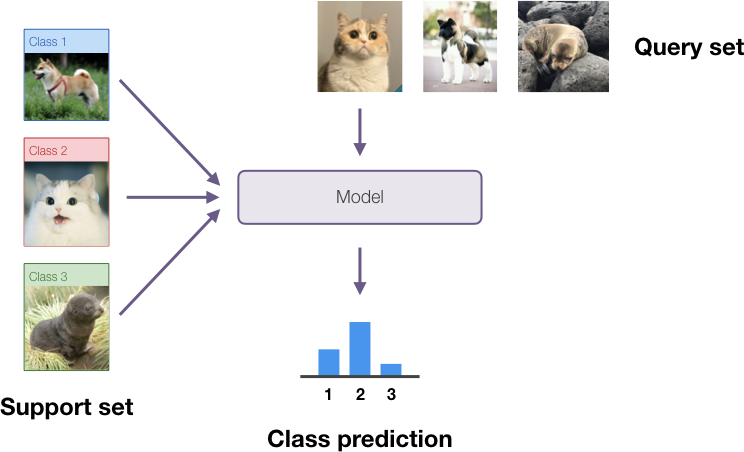In this setting we assume we have 1 to 10 training data points per class. This amount of data is too small to realistically perform gradient descent on when a model has a very large number of parameters, and this led to the creation of a different class of methods dedicated to solving this problem. In this setting we consider the dataset to be split into two parts: the support set for which we have both inputs and outputs (target classes); and the query set for which we have only the inputs. When not using pretrained models, the training dataset is split into a number of support/query sets for training (sometimes called meta-training) and others for testing. In this case some of the classes are held out from the meta-training dataset so that there is some novelty.

## Main methods for few shot classification

The most popular approaches to tackle this problem can be split into two broad categories, which I’ll outline below.

The first approach is to map the input data to a high dimensional representation space via a neural network backbone trained on other train/test data splits (in the case of image classification, often a convolutional network). On this representation space, an adaptation method can be used to split the data into different volumes corresponding to the different classes based on the support set labels.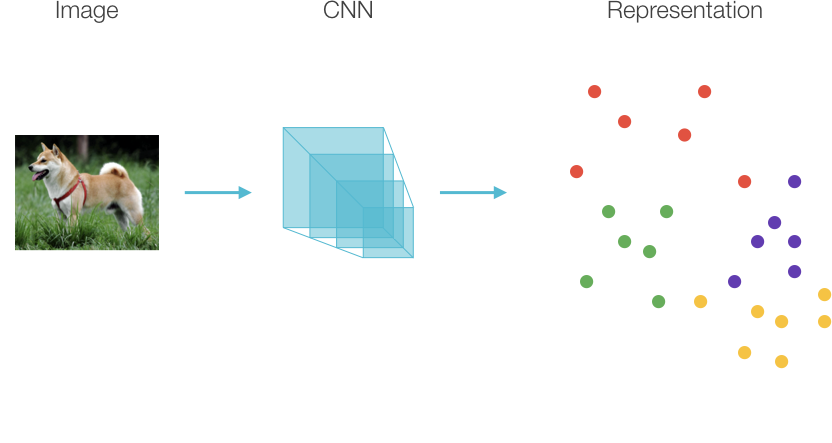Earliest work in this branch is [Snell17] which creates clusters by averaging all representations in a given class in the support set and then uses a distance metric to calculate posterior class probabilities; and [Vinyals16] which creates a KDE estimate of the posterior class probability distribution based on the distance of the test point to its k-nearest neighbors. Performance for these methods is largely reliant on the representations created by the neural network backbone and results keep improving as bigger and deeper models are deployed.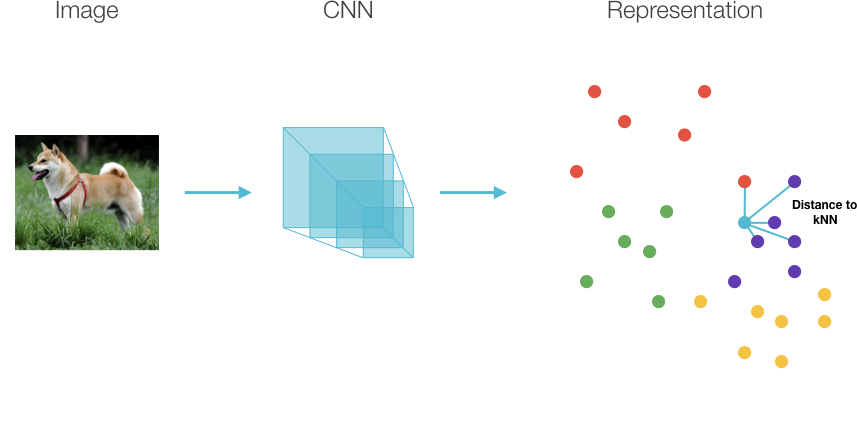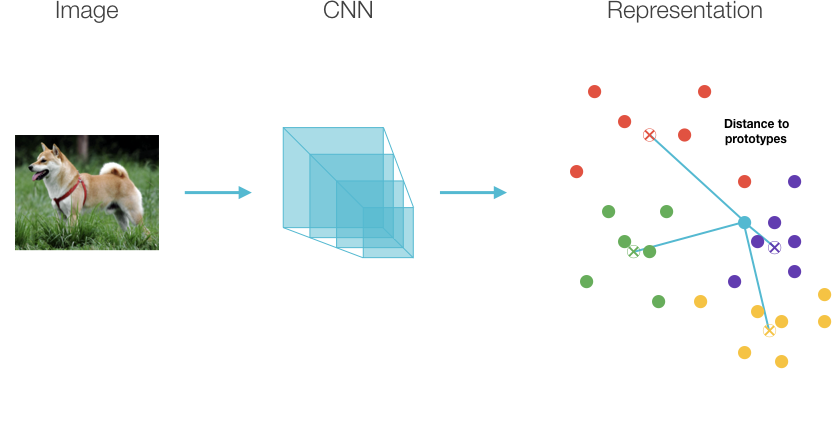Another approach is to find a more efficient way to adapt the parameters of a classifier than through standard gradient descent alone. Early work [Ravi17] trained an LSTM to calculate the weight updates of a standard convolutional network, instead of using traditional gradient descent. The hope here is that the LSTM learns a good prior for what the weight updates should look like, given the query set.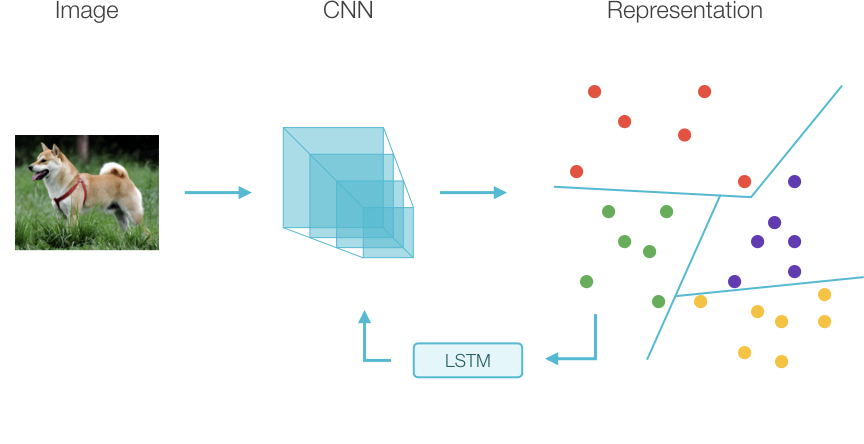An idea with a very similar spirit was proposed in [Finn17] with MAML, one of the most popular few-shot classification algorithms currently. The authors propose training a network using two nested optimization loops: for each support/query set we have available for training, we initialize the network to the current set of parameters and perform a few steps of standard gradient descent over the cross entropy classification loss. Then we can backpropagate through that whole optimization to take one step of gradient descent over the initialization parameters to find a ‘good’ initialization with which we can get good performance after few steps of gradient descent on all train/test datasets.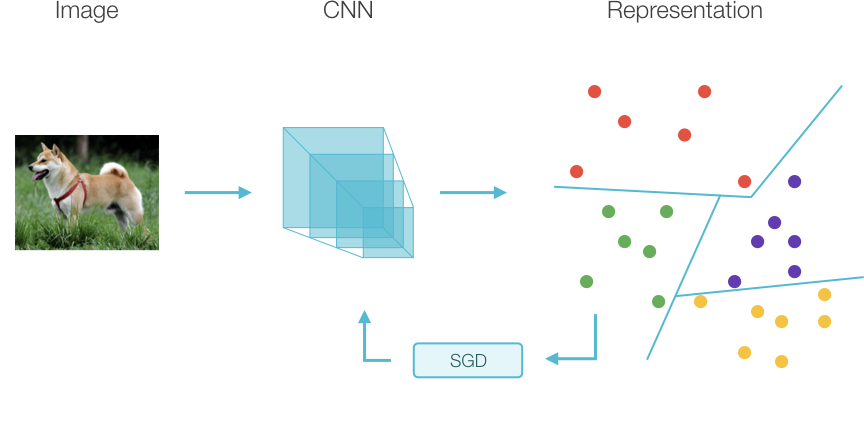A big issue with MAML is that it is computationally expensive as it requires the calculation of second derivatives, and numerous approximations have been proposed to alleviate this. Recent work [Raghu19] has shown that even for full MAML the ‘inner’ optimization loop (the few steps of vanilla gradient descent on the support set) are doing little more than changing the weights of the final classification head. The authors thus propose replacing the MAML update with fine-tuning of the final classification layer, which brings us back to the fixed representation extractor paradigm.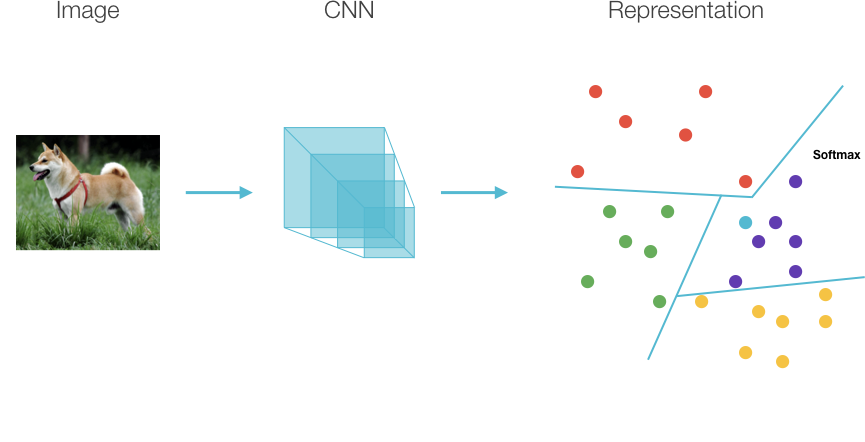While it is never explicitly mentioned in most literature, there is a large overlap in concerns between transfer learning and few-shot learning, as recent papers have shown that to achieve good results with deep learning models in few-shot classification we have to effectively leverage some kind of prior knowledge. In the image below I summarize my current understanding of the techniques broadly applicable to a problem as a function of degree of domain transfer possible and in-domain data size.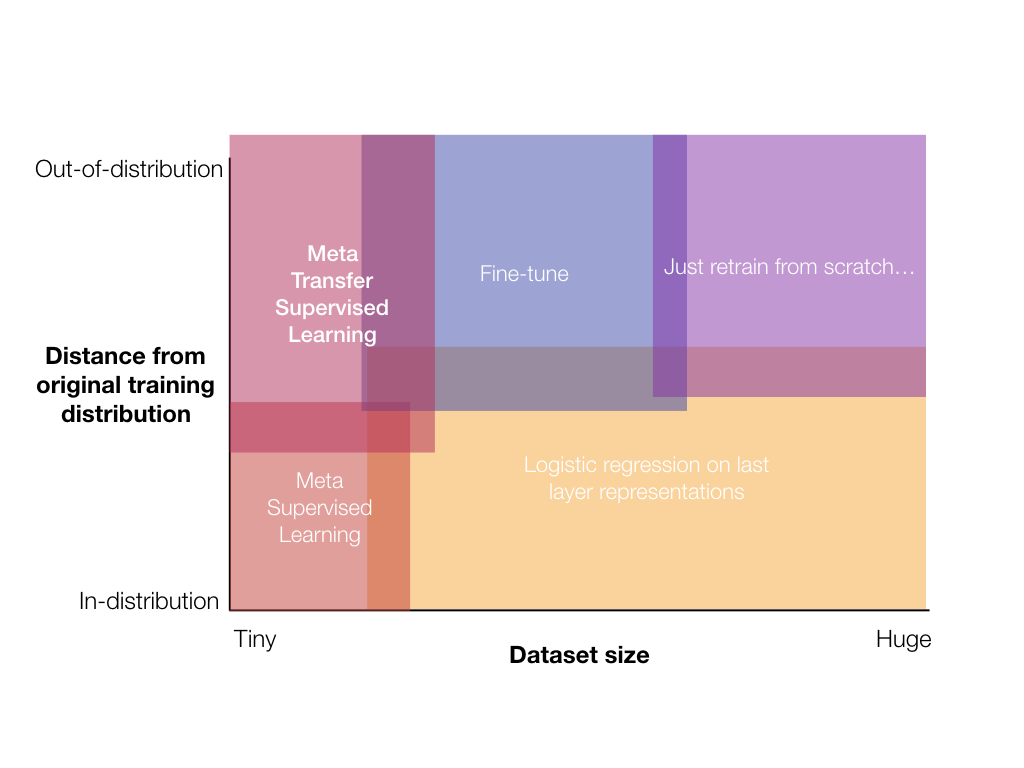Starting from the easiest problems, if you have a large dataset for your support set you often want to just train your model from scratch. If your dataset is significantly in-domain, you can probably get away with just retraining the classification head (i.e. logistic regression - the orange area in the schematic). Let’s quickly review transfer learning before continuing.

## Transfer learning

When you have a bit less data, you can either fine-tune your whole model or just retrain the classification layer. A number of papers have investigated the tradeoffs for this scenario [Zhai19] [Kornblith18] concluding that it’s all about the representations:

• Networks pretrained on ImageNet transfer well to natural image datasets, with transfer performance positively correlated with original performance on ImageNet.
• Those networks however, transfer poorly to artificial datasets or highly fine-grained tasks, where discriminative features are probably not encoded in the networks’ representations.
• Networks trained with self-supervision seem to have a performance boost, while networks trained in a fully unsupervised way don’t really transfer well. Again this points to the original supervision signal forcing the network to encode discriminative features in the final representation.

There are significant caveats to using transfer learning with networks trained on ImageNet though. We know that their generalization power is quite brittle and very dependent on the dataset’s specific data distribution [Recht19], which could imply the representations are not as robust as we would like them to be.

A lot of recent work points towards ways to improve representation genrality such as training with adversarial robustness [Engstrom19], network architecture changes [Gilboa19], and training with more data / data augmentation [Xie19].

## Transfer few-shot classification

Early work in few shot classification disregarded the transfer aspect at all. Training was performed on the meta training dataset with some classes held out, and performance was tested on remaining in-distribution held out classes. While this tests one aspect of generalization, it doesn’t tell us much about few-shot cross-domain transfer generalization. [Triantafillou19] looked at cross-domain transfer in the few-shot setting and found generally weak performance for all methods when tested on out-of-domain datasets. Training across a wider variety of datasets seems to improve performance, which hints at the fact that, again, it’s all about the representations.

In fact [Chen19] very explicitly demonstrates that few-shot classification accuracy is much more strongly correlated with backbone parameter count and depth (indirectly a proxy for representation quality) than with a specific few-shot adaptation method, and [Dhillon19] finds that even last layer fine-tuning can be competitive with few-shot classification methods when initialized properly. All this evidence points to the fact that few-shot classification is currently limited by representation generality and robustness.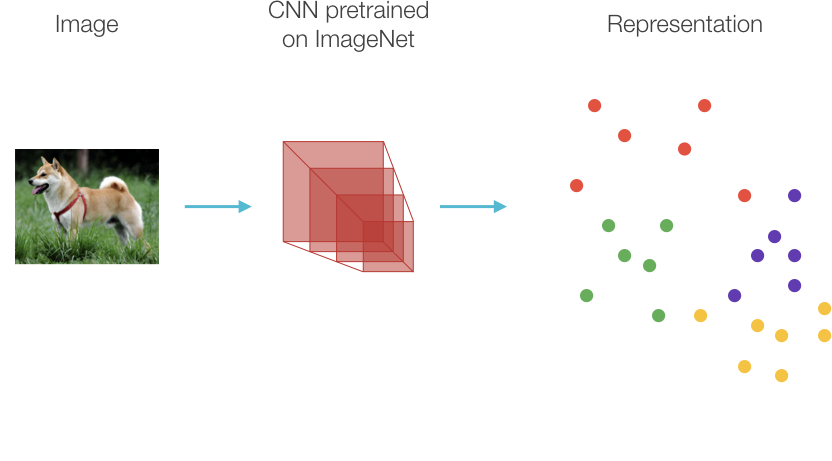In our recent work [Ramalho19] we investigate the performance of directly using CNNs pretrained on Imagenet as the feature backbone and plugging those representations into various adaptation methods. Since previous lines of research have shown that: bigger models are better, and representation quality is mostly what matters; then we want to look at what happens when we take the biggest models trained on the largest image dataset and test their transfer performance on few-shot classification tasks. The key takeaways of our investigation is as follows:

• Bigger models with better classification performance on ImageNet do perform better for few-shot classification when the tasks are not too out-of-domain (i.e. natural images, object classification tasks). If the tasks are more fine-grained, performance suffers but classification models still do well. For totally out-of-domain datasets, their performance tanks. Below you can see average accuracy for EfficientNetB7 for each dataset. Fine grained classification tasks such as cars, planes, funghi, and svhn all could use serious improvement.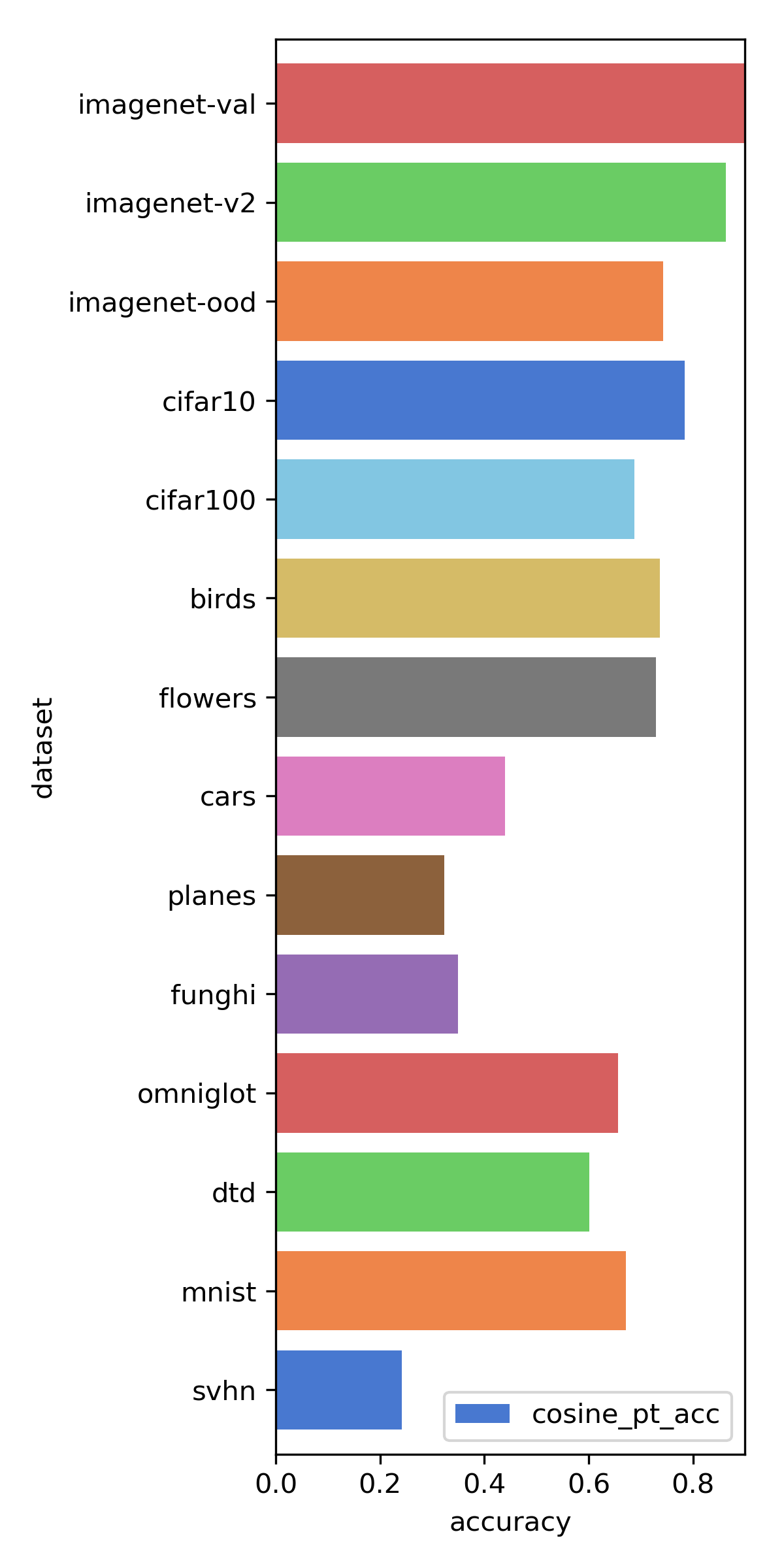• Unsupervised models’ representations aren’t really better even when considering cross-domain transfer. We’d hoped that these models’ representation spaces would be less overfit to the ImageNet classification task and therefore transfer better, but that does not seem to be the case.

• Models trained with robustness transfer a bit better, but not enough to really matter.

• Prototype networks work for the backbones we tested. And best if we choose cosine distance instead of euclidean (models originally trained with a softmax classification objective try to maximize the dot product between the vector corresponding to a specific class and the representation of the current point, which induces a specific geometry in representation space better captured by cosine distance). Below I plotted the average accuracy over all datasets and all backbones for 5 shots classification. You can see that Prototype Networks is slightly better than Matching Networks for all distance functions, and cosine distance is better than L2 and dot product.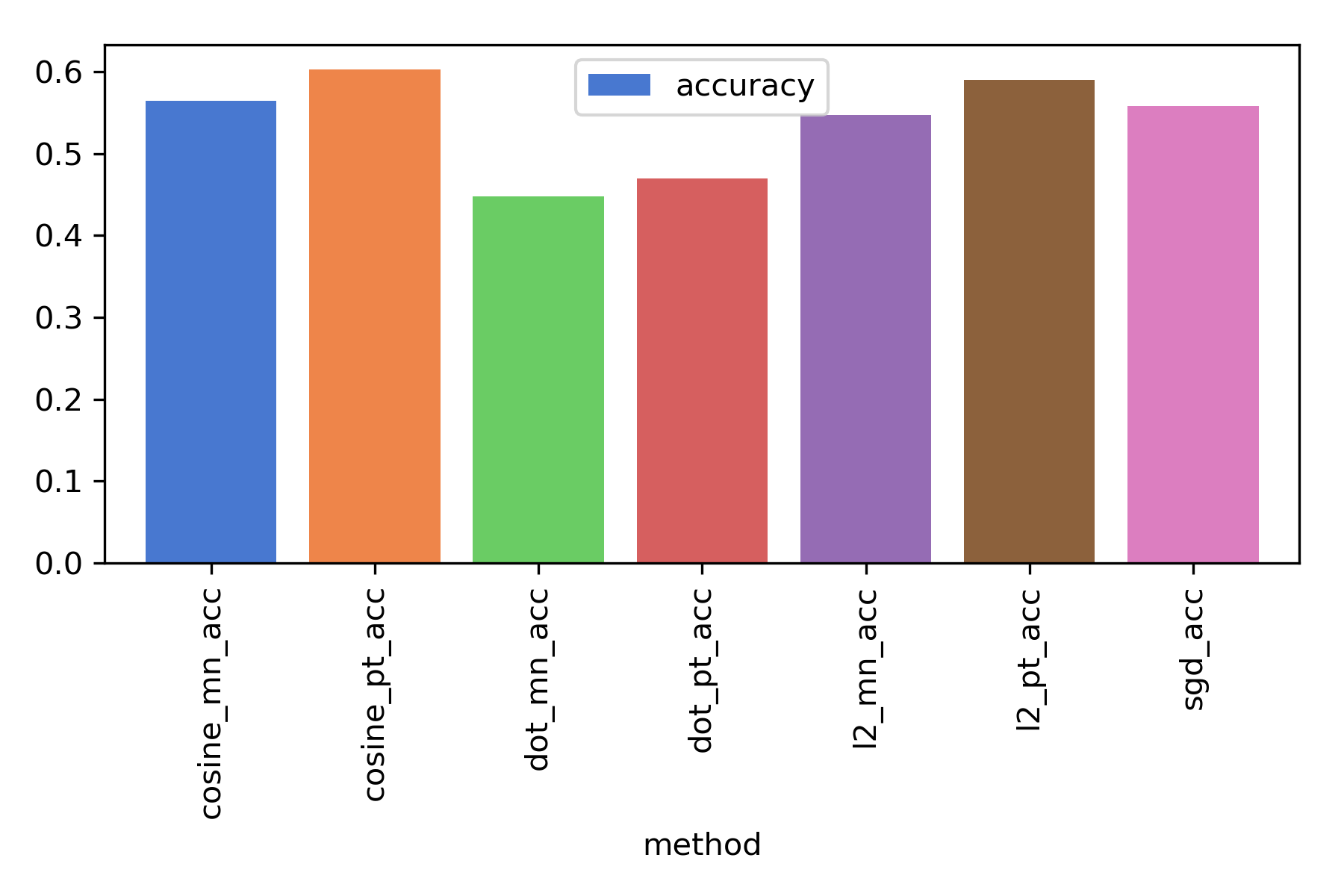• For 10 samples and above, just finetune the classification layer instead of using few-shot classification methods! You can see a summary of the accuracy as a function of number of shots below comparing Prototype Networks with Logistic Regression with SGD on the last layer (for the EfficientNetB7 backbone).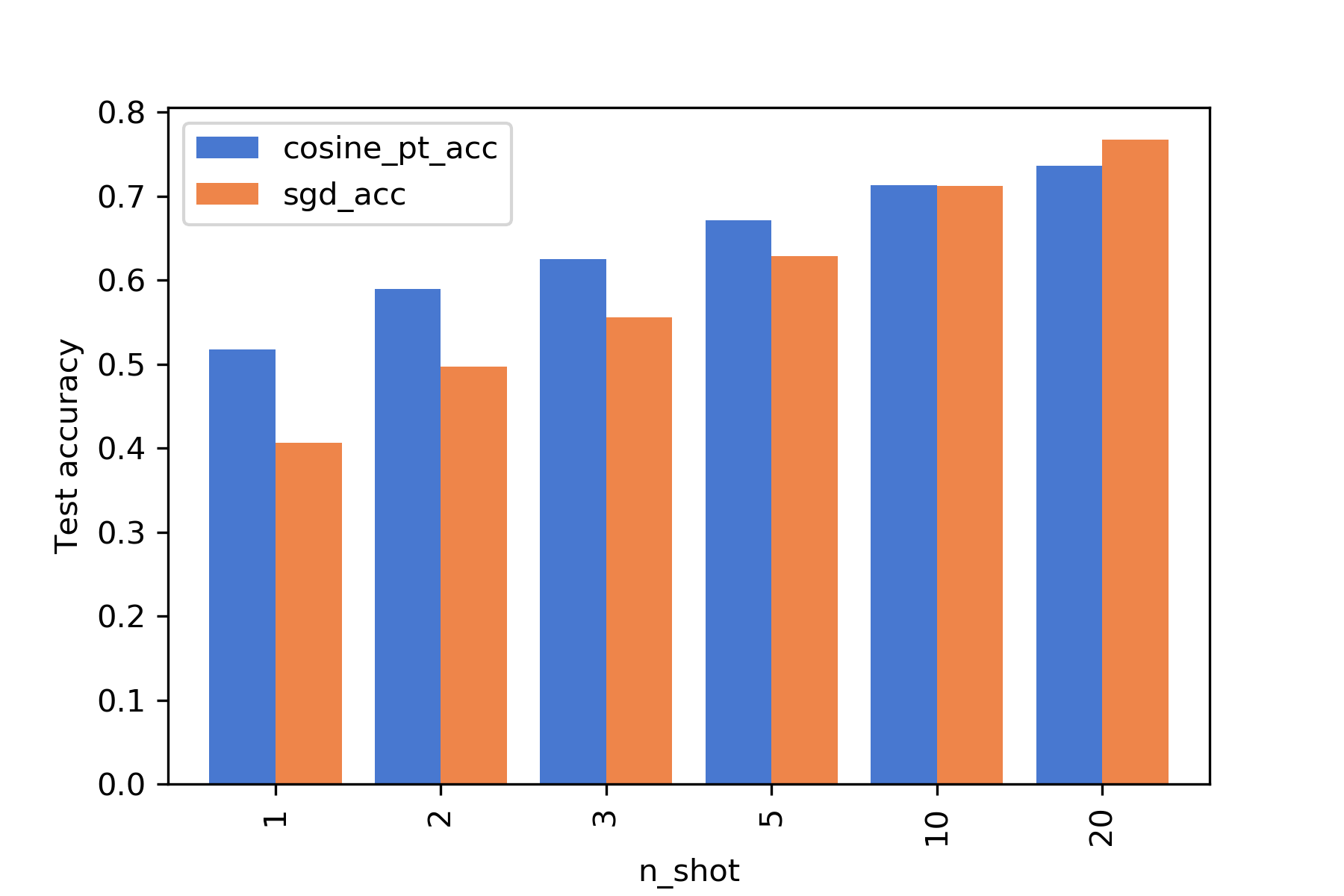# Dimensionality reduction 101: linear algebra, hidden variables and generative models

Suppose you are faced with a high dimensional dataset and want to find some structure in the data: often there are only a few causes, but lots of different data points are generated due to noise corruption. How can we infer these causes? Here I’m going to cover the simplest method to do this inference: we will assume the data is generated by a linear transformation of the hidden causes. In this case, it is quite simple to recover the parameters of this transformation and therefore determine the hidden (or latent) variables which represent their cause.

Mathematically, let’s say the data is given by a high-dimensional (size N) vector $u$ which is generated by a low-dimensional (size M) vector $v$ via

$$u=\sum_i^M W_i v_i + \text{noise}$$

where $W_i$ are N-dimensional vectors which encode the transformation. For simplicity I will assume $u$ to have mean value 0. Let’s assume the noise is gaussian. Then the distribution for the data is

$$P(u|v) = \frac{1}{\sqrt{(2\pi)^N\left|\Sigma\right|}}\exp\left(-\frac{1}{2}(u-W\cdot v)^T \Sigma^{-1}(u-W\cdot v)\right)$$

with $\Sigma$ a diagonal matrix. This means that we assume any correlation structure in the data arises from the transformation $W$, and not due to noise correlations. This model is the main feature of the procedure known as Factor analysis.

To fit the model we need both the weights $W$ and factors $v$ which best fit the data (in the case of no prior information for $v$, this corresponds to maximising the likelihood function). It is convenient to take the logarithm of the likelihood function:

$$\log P(u|v) = -\frac{1}{2}(u-W\cdot v)^T \Sigma^{-1}(u-W\cdot v) -\frac{1}{2} \log |\Sigma| - \frac{N}{2} \log 2\pi$$

So we are looking for $\arg\max_{W,v,\Sigma} \log P(u|v)$. The obvious way to obtain the parameters is to use expectation-maximisation: we calculate what are the factors given $W$ and $\Sigma$, and then update those parameters given the new factors in such a way as to maximise the log-likelihood. Iterating this process will result in convergence to a final set of weights and factors, which will be a local minimum of the likelihood.

Let’s examine how this works with a very simple example: the Iris dataset. This is a 4 dimensional dataset, with each dimension corresponding to a physical measurement of the sepals and petals of a flower. There are 3 different flowers so we can assume that each flower’s genome will code for different physical features. So the question is whether there is a ‘genome variable’ $v$ which maps into the observed physical features $u$? Let’s load the dataset directly using sklearn:

from sklearn import datasets

x = iris.data # physical measurements
y = iris.target # actual species for visualization


And now we can plug this directly into the Factor analysis model:

import matplotlib.pyplot as plt
from sklearn.decomposition import FactorAnalysis

model = FactorAnalysis(n_components=2)
x_reduced = model.fit_transform(x)
plt.scatter(x_reduced[:, 0], x_reduced[:, 1], c=y, cmap=plt.cm.Paired)


Let’s visualize the hidden factors for the actual data points we have by inverting the transformation. Below you can see the data with $v_1$ in the x-axis and $v_2$ in the y-axis.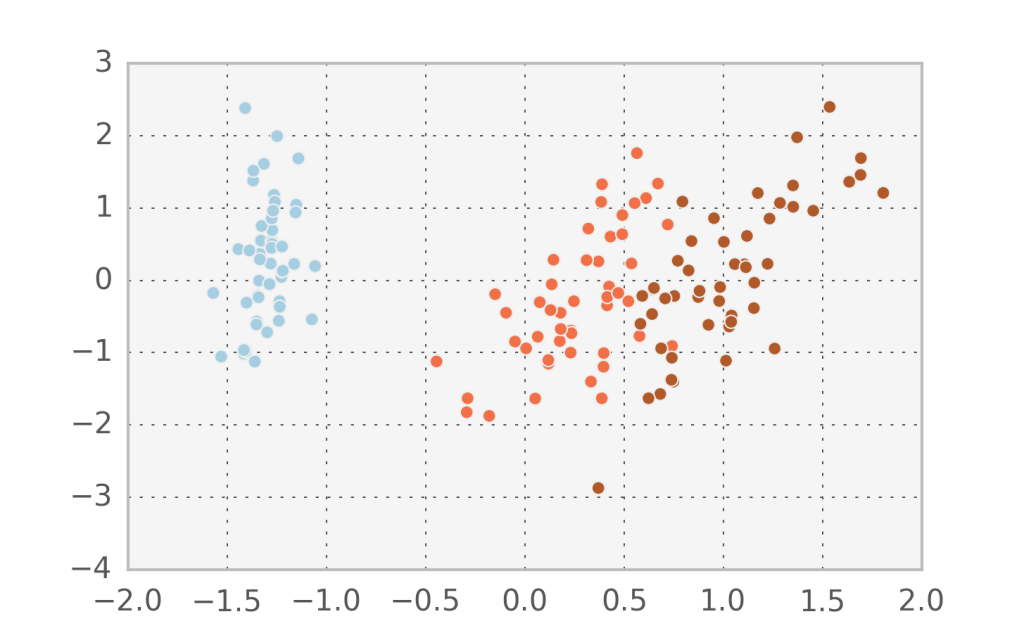In fact, it seems that the first component $v_1$ manages to describe accurately the variation between the 3 different species. Let’s try to do Factor analysis with just one component and see what comes out (I added some randomness in the y-direction to visualize the points better).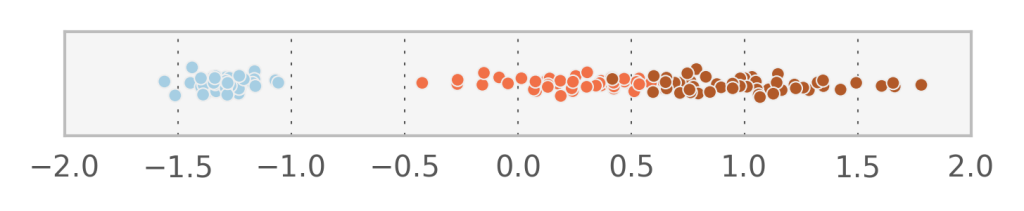With 1 component we obtain an estimate for $\Sigma^{-1} = [ 0.16350346, 0.15436415, 0.00938691, 0.04101233]$ which corresponds to the variation of the data which is not explained by the linear transformation of $v$. What if we would force all diagonal elements of $\Sigma$ to be identical? In that case, we observe that the vectors $W_i$ actually form an orthogonal basis (i.e. each one is perpendicular to the next). This in turn means that when we project the data to the space of the hidden variables, each $v_i$ will be uncorrelated to the others (which does not happen with Factor analysis).

Linear algebra explains why this happens: after some calculation, we can observe that the obtained $W_i$ correspond to the principal eigenvectors (i.e. are associated to the largest eigenvalues) of the sample covariance matrix $C= \frac{1}{N}\sum_{j=1}^N (u^j - \bar{u}) (u^j-\bar{u})^T$ with the overscripts denoting sample index. This method has a specific name and is called Probabilistic Principal Components Analysis. Setting $\Sigma = 0$ we obtain the popular vanilla PCA.

Typically we sort the eigenvectors such that $W_1$ corresponds to the largest eigenvalue of $C$ meaning that the hidden variable $v_1$ explains the most variation in the observed data. Now $W_2$ must be perpendicular to $W_1$, which means that $v_2$ will explain the most remaining variation in the data, and so on. If we allow $M=N$ then it would be possible to completely reconstruct the data from $v$.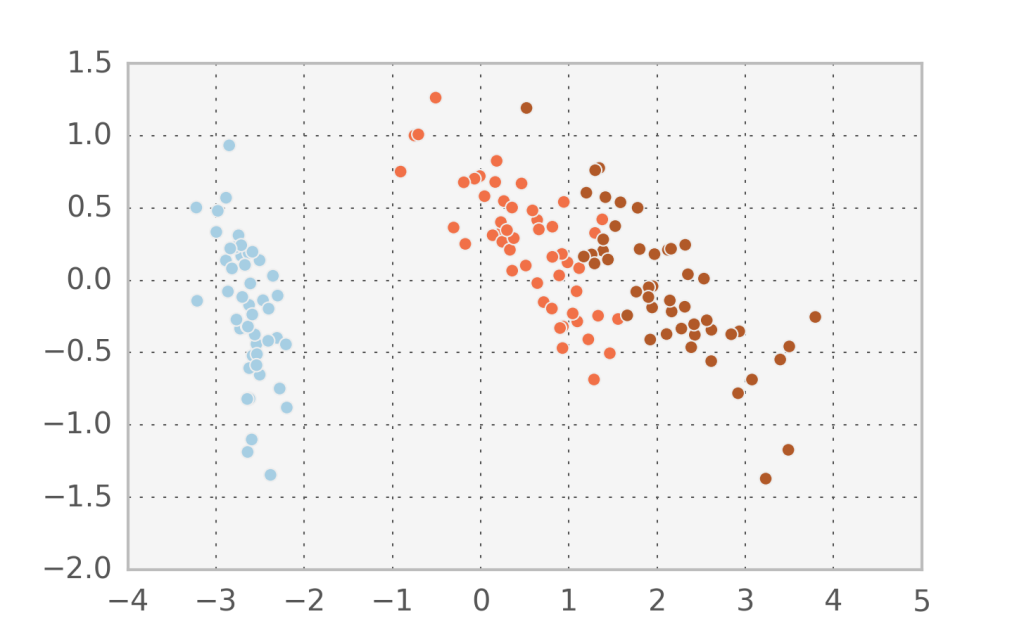In the Iris dataset we can observe that the projected subspace using probabilistic PCA is different to the one found in Factor analysis. We can confirm that we found orthogonal components by printing $W_1 \cdot W_2$ and comparing it to Factor analysis:

model = PCA(n_components=2)
x_reduced = model.fit_transform(x)
print np.dot(model.components_, model.components_)
# 3.43475248243e-16

model = FactorAnalysis(n_components=2)
x_reduced = model.fit_transform(x)
print np.dot(model.components_, model.components_)
# 0.146832660483


In the case of handwritten digits, the first two components in PCA appear to capture more of the structure of the problem than the first two factors in factor analysis. However, since this is a strongly nonlinear problem, none of them can accurately separate all 10 digit classes.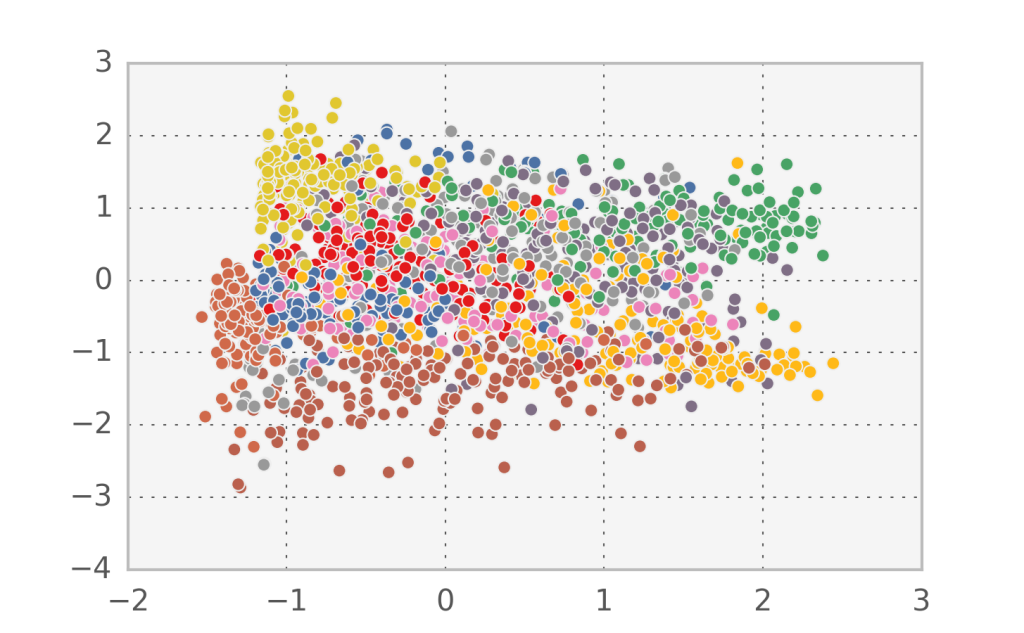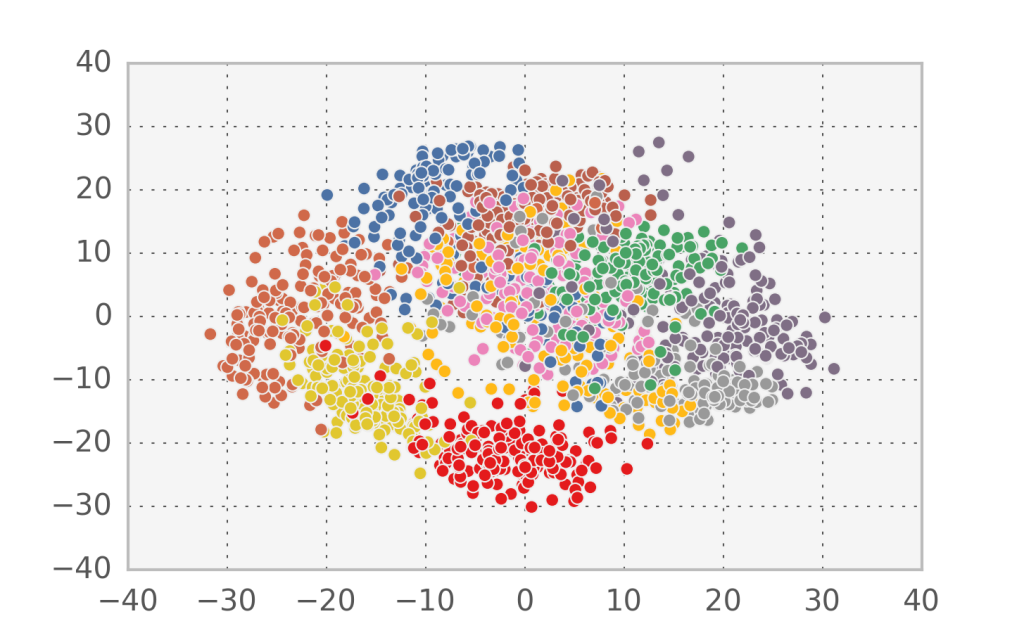If we look at the log-likelihood equivalent for PCA we observe a very deep connection: $\log P(u|v) = -\frac{1}{2}(u-W\cdot v)^2$. The $W, v$ that come out of PCA (i.e. maximise the log-likelihood) also minimize the least squares reconstruction error for the simplest linear model. From a data compression perspective, that means that we can compress the information contained in $u$ by saving only $W$ and $v$. This is worth it when we have a large number of samples of $u$, since $W$ is constant and each $v$ is smaller than each $u$.

With the fit distribution in hand, we can even generate new samples of $u$. We just need to draw some samples of low-dimensional $v$ and pass it through the linear model we have. Let’s see what happens when we apply this idea to the digits dataset, sampling $v$ from a gaussian approximation of the $v$ corresponding to the actual data distribution.

v = model.transform(x)
v_samples = np.random.multivariate_normal(
np.mean(v, axis=0), np.cov(v.T), size=100)
x_samples = model.inverse_transform(v_samples)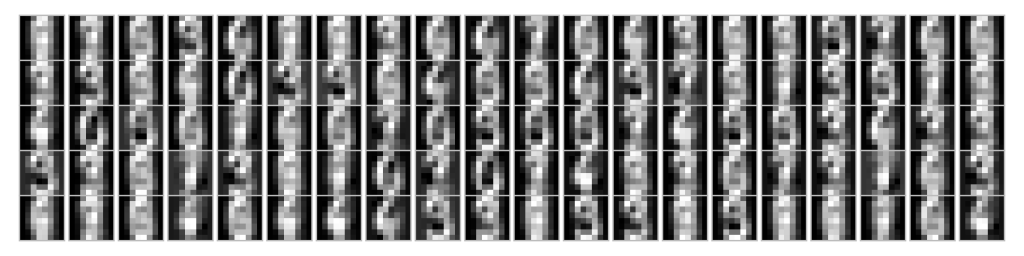To finish this long post, let’s just look at what happens if we don’t assume a flat prior for $v$. Two common options are to assume $P(v)$ is a gaussian or an exponential distribution. Then the log-likelihood (getting rid of constants, as usual) becomes:

$$\log P(u|v) = -\frac{1}{2}(u-W\cdot v)^2 - \alpha v^2 - \beta |v|$$

Those two terms correspond to the often used $L_2$ and $L_1$ regularization, which for linear models have the fancy names of ridge regression and lasso respectively (not really sure why they need fancy names). Writing this in a more CS notation, we can say that our problem consists of maximising:

$$||u-W\cdot v||^2 - \alpha ||v||^2 - \beta ||v||$$

This problem has been given the name of Sparse PCA, and we can find an implementation in scikits. Let’s see what happens when we apply it to the digits dataset.

from sklearn.decomposition import SparsePCA
from sklearn import datasets

x = dset.data
y = dset.target

model = SparsePCA(n_components=10, alpha=0)
x_reduced = model.fit_transform(x)

print np.dot(model.components_, model.components_)

n_row = 5
n_col = 2
plt.figure(figsize=(6, 15))
for i in xrange(10):
comp = model.components_[i]
plt.subplot(n_row, n_col, i + 1)
vmax = max(comp.max(), -comp.min())
plt.imshow(comp.reshape([8,8]), cmap=plt.cm.gray, interpolation='nearest')
plt.xticks(())
plt.yticks(())
plt.tight_layout()
plt.savefig('digits_SPCA_rec.png')


Immediately we notice that we lose the orthogonality of the components due to the constraints. Let’s see how they look like: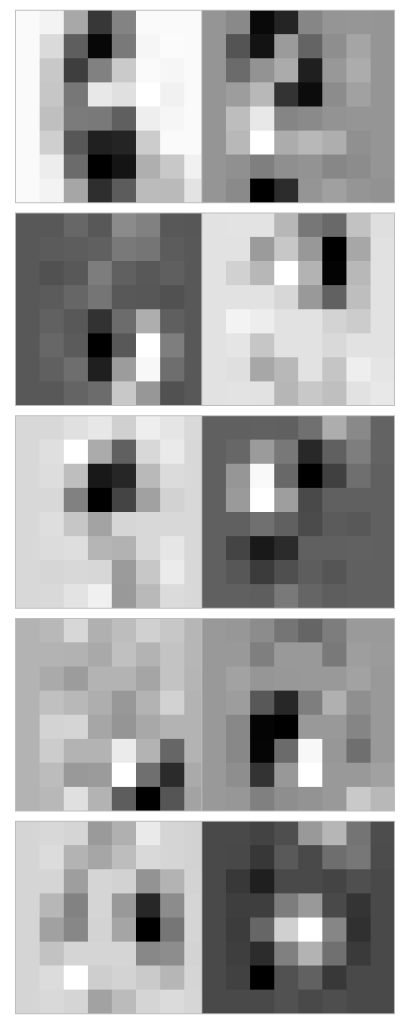Here is a gist with all the code used for this post.

# Parallel programming with opencl and python: parallel scan

This post will continue the subject of how to implement common algorithms in a parallel processor, which I started to discuss here. Today we come to the second pattern, the scan. An example is the cumulative sum, where you iterate over an array and calculate the sum of all elements up to the current one. Like reduce, the algorithm we’ll talk about is not exclusive for the sum operation, but for any binary associative operation (the max is another common example). There are two ways to do a parallel scan:  the hills steele scan, which needs $\log n$ steps; and the blelloch scan, requiring $2 \log n$ steps. The blelloch scan is useful if you have more data than processors, because it only needs to do $\mathcal{O}(n)$ operations (this quantity is also referred to as the work efficiency); while the hillis steele scan needs $\mathcal{O}(n \log n)$ operations. So let’s look at how to implement both of them with opencl kernels.

#### Hillis Steele

This is the simplest scan to implement, and is also quite simple to understand. It is worthwhile noting that this is an inclusive scan, meaning the first element of the output array o is described as a function of the input array a as follows: $o_i = \sum_{j=0}^i a_j$

Naturally, you could replace the summation with any appropriate operator. Rather than describing the algorithm with words, I think a picture will make it much clearer than I could: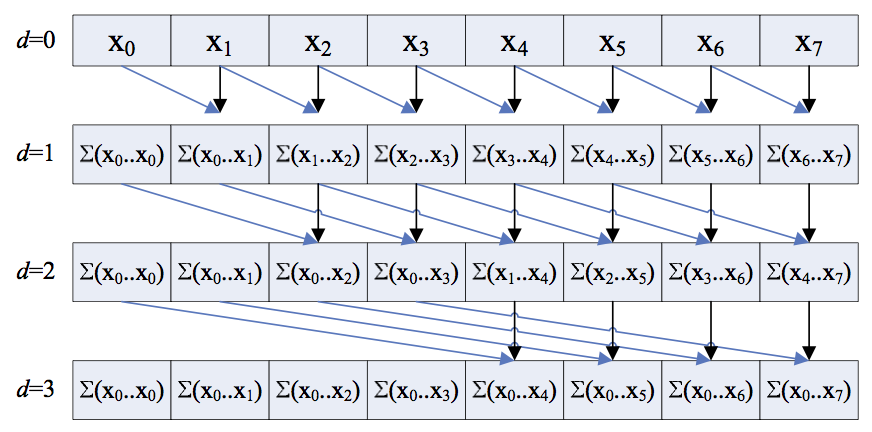Visualization of the hillis steele inclusive scan. Credit: nvidia.

As you can see, the key is to add the value of your neighbor $2^d$ positions to your left to yourself, or just do nothing if such neighbor does not exist. This is quite simple to translate to an OpenCL kernel:

#define SWAP(a,b) {__local float *tmp=a;a=b;b=tmp;}
__kernel void scan(__global float *a,
__global float *r,
__local float *b,
__local float *c)
{
uint gid = get_global_id(0);
uint lid = get_local_id(0);
uint gs = get_local_size(0);

c[lid] = b[lid] = a[gid];
barrier(CLK_LOCAL_MEM_FENCE);

for(uint s = 1; s &lt; gs; s &lt;&lt;= 1) {
if(lid &gt; (s-1)) {
c[lid] = b[lid]+b[lid-s];
} else {
c[lid] = b[lid];
}
barrier(CLK_LOCAL_MEM_FENCE);
SWAP(b,c);
}
r[gid] = b[lid];
}


The for loop variable s represents the neighbor distance: it is multiplied by two every loop until we reach N neighbors. Note the use of the SWAP macro: it swaps the b and c pointers which will always denote the current step (c) and the previous step (b) without needing to copy memory.

#### Blelloch

The Blelloch scan is an exclusive scan, which means the sum is computed up to the current element but excluding it. In practice it means the result is the same as the inclusive scan, but shifted by one position to the right: $o_i = \sum_{j=0}^{i-1} a_j,o_0=0$

The idea of the algorithm is to avoid repeating summations of the same numbers. As an example, if you look at the picture above you can see that to calculate $o_5$ we need to add together ${x_5, x_4, x_2+x_3, x_1+x_0}$. That means we are essentially repeating the calculation of $o_3$ for nothing. So to avoid this we’d need to come up with a non overlapping set of partial sums that each calculation could reuse. That’s what this algorithm does!

As before, the algorithm is better explained with a picture or two: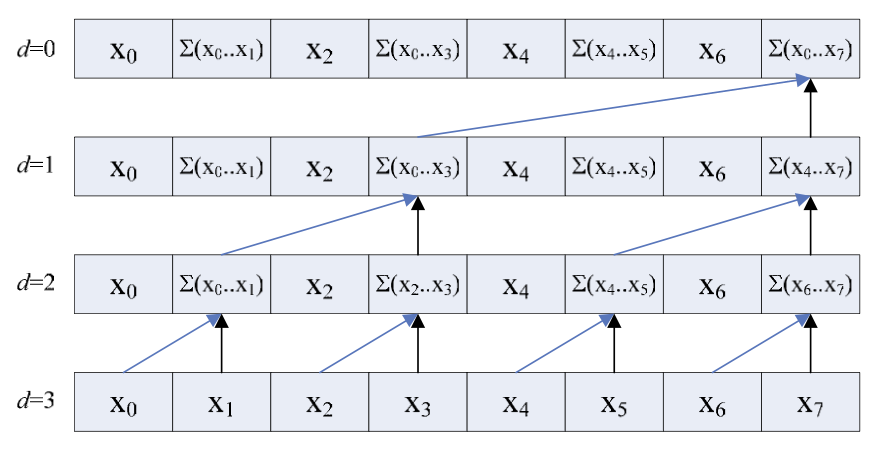The up sweep portion of the Blelloch scan. Here the partial sums are calculated. Credit: nvidia.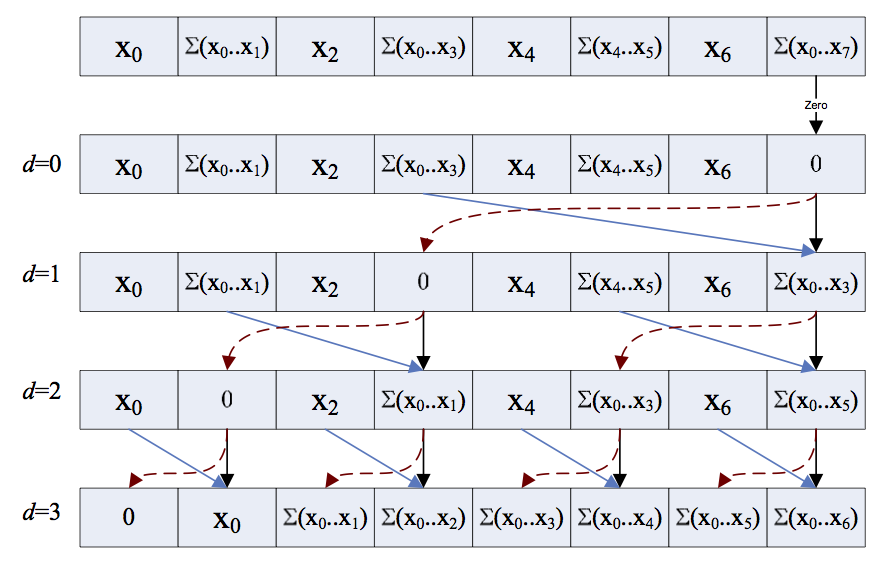The down sweep portion of the Blelloch scan. Here the partial sums are used to calculate the final answer.

You can see how the up sweep part consists of calculating partial sums, and the down sweep combines them in such a way that we end up with the correct results in the correct memory positions. Let’s see how to do this in OpenCL:

__kernel void scan(__global float *a,
__global float *r,
__local float *b,
uint n_items)
{
uint gid = get_global_id(0);
uint lid = get_local_id(0);
uint dp = 1;

b[2*lid] = a[2*gid];
b[2*lid+1] = a[2*gid+1];

for(uint s = n_items&gt;&gt;1; s &gt; 0; s &gt;&gt;= 1) {
barrier(CLK_LOCAL_MEM_FENCE);
if(lid &lt; s) {
uint i = dp*(2*lid+1)-1;
uint j = dp*(2*lid+2)-1;
b[j] += b[i];
}

dp &lt;&lt;= 1;
}

if(lid == 0) b[n_items - 1] = 0;

for(uint s = 1; s &lt; n_items; s &lt;&lt;= 1) {
dp &gt;&gt;= 1;
barrier(CLK_LOCAL_MEM_FENCE);

if(lid &lt; s) {
uint i = dp*(2*lid+1)-1;
uint j = dp*(2*lid+2)-1;

float t = b[j];
b[j] += b[i];
b[i] = t;
}
}

barrier(CLK_LOCAL_MEM_FENCE);

r[2*gid] = b[2*lid];
r[2*gid+1] = b[2*lid+1];
}


It took me a little while to wrap my head around both steps of the algorithm, but the end code is pretty similar to the Hillis Steele. There are two loops instead of one, and the indexing is a bit tricky, but I think that comparing the code to the picture it should be straightforward. You can also find a cuda version in the nvidia paper where I took the pictures from.

# Parallel programming with opencl and python: parallel reduce

Once you know how to use python to run opencl kernels on your device (read Part I and Part II of this series) you need to start thinking about the programming patterns you will use. While many tasks are inherently parallel (like calculating the value of a function for N different values) and you can just straightforwardly run N copies on your processors, most interesting tasks involve dependencies in the data. For instance if you want to simply sum N numbers in the simplest possible way, the thread doing the summing needs to know about all N numbers, so you can only run one thread, leaving most of your cores unused. So what we need to come up with are clever ways to decompose the problem into individual parts which can be run in parallel, and then combine them all in the end.

This is the strong point of Intro to Parallel Programming, available free. If you really want to learn about this topic in depth you should watch the whole course. Here I will only show how I implemented some of the algorithms discussed there in OpenCL (the course is in CUDA). I’ll discuss three algorithms: reduce, scan and histogram. They show how you can use some properties of mathematical operators to decompose a long operation into a series of many small, independent operations.

Reduce is the simplest of the three. Let’s say you have to sum N numbers. On a serial computer, you’d create a temporary variable and add the value of each number to it in turn. It appears there is not much to parallelize here. The key is that addition is a binary associative operator. That means that $a + b + c + d = (a+b) + (c+d)$. So if I have two cores I can add the first two numbers on one, the last two on the other, and then sum those two intermediate results. You can convince yourself that we only need $\mathcal{O}(\log N)$ steps if we have enough parallel processing units; as opposed to $\mathcal{O}(N)$ steps in the serial case. A reduction kernel in OpenCL is straightforward, assuming N is smaller than the number of cores in a single compute unit:

__kernel void reduce(__global float *a,
__global float *r,
__local float *b)
{
uint gid = get_global_id(0);
uint wid = get_group_id(0);
uint lid = get_local_id(0);
uint gs = get_local_size(0);

b[lid] = a[gid];
barrier(CLK_LOCAL_MEM_FENCE);

for(uint s = gs/2; s &gt; 0; s &gt;&gt;= 1) {
if(lid &lt; s) {
b[lid] += b[lid+s];
}
barrier(CLK_LOCAL_MEM_FENCE);
}
if(lid == 0) r[wid] = b[lid];
}


Full code here. First we copy the numbers into shared memory. To make sure we always access memory in a contiguous fashion, we then take the first N/2 numbers and add to them the other N/2, so now we have N/2 numbers to add up. Then we take half of those and add the next half, until there is only one number remaining. That’s the one we copy back to main memory.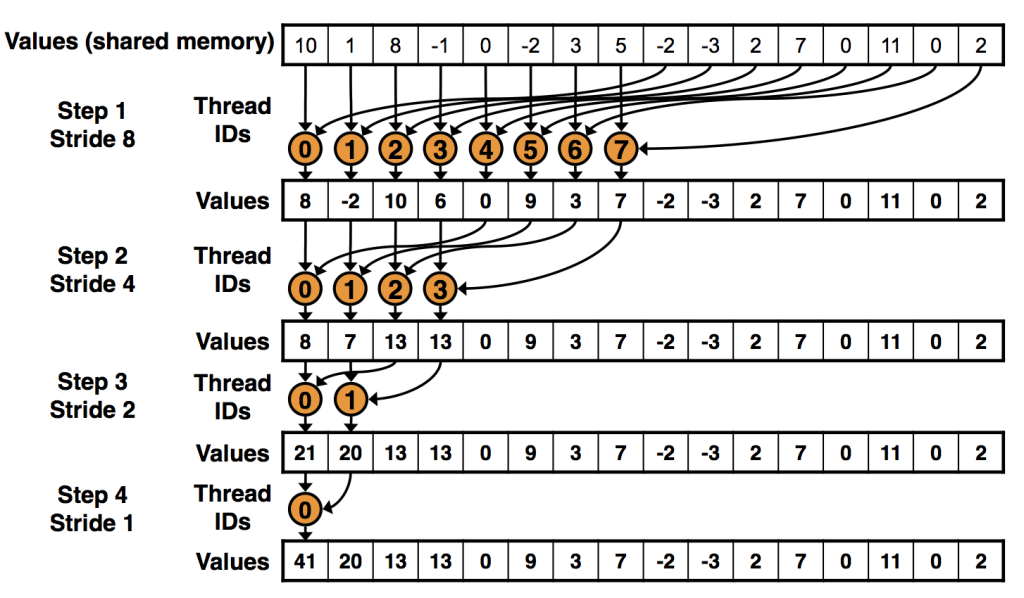Reduction stages. Image from nvidia slides.

If we have N bigger than the number of cores in a single unit, we need to call the kernel multiple times. Each core will compute its final answer, and then we run reduce again on that array of answers until we have our final number. In the python code I linked to, you can see how we enqueue the kernel twice, but with fewer threads the second time:

'''run kernels the first time for all N numbers, this will result
in N/n_threads numbers left to sum'''
evt = prg.reduce(queue, (N,), (n_threads,), a_buf, r_buf, loc_buf)
evt.wait()
print evt.profile.end - evt.profile.start

left to sum, we sum those here, leaving 1 number'''
evt.wait()
print evt.profile.end - evt.profile.start

'''copy the scalar back to host memory'''
cl.enqueue_copy(queue, o, o_buf)


# Parallel programming with opencl and python: vectors and concurrency

Last time we saw how to run some simple code on the gpu. Now let’s look at some particular aspects related to parallel programming we should be aware of. Since gpus are massively parallel processors, you’d expect you could write your kernel code for a single data piece, and by running enough copies of the kernel you’d be maximizing your device’s performance. Well, you’d be wrong! I’m going to focus on the three most obvious issues which could hamper your parallel code’s performance:

• Each of the individual cores is actually a vector processor, which means it can perform an operation on multiple numbers at a time.
• At some point the individual threads might need to write to the same position in memory (i.e. to accumulate a value). To make sure the result is correct, they need to take turns doing it, which means they spend time waiting for each other doing nothing.
• Most code is limited by memory bandwidth, not compute performance. This means that the gpu can’t get the data to the processing cores as fast as they can actually perform the computation required.

Let’s look at the vector instructions first. Let’s say you need to add a bunch of floating point numbers. You could have each core add one number at a time, but in reality you can do better. Each processing core can actually perform that addition on multiple numbers at a time (currently it is common to process the equivalent of 4 floats at a time, or a 128 bit vector). You can determine the maximum size of a vector for each type by accessing the CL_DEVICE_PREFERRED_VECTOR_WIDTH_ [property](http://documen.tician.de/pyopencl/runtime.html) for a device.

To take advantage of this feature, openCL defines types such as float4, int2 etc with overloaded math operations. Let’s take a look at a map operation implementing vectors:

__kernel void sq(__global float4 *a,
__global float *c)
{
int gid = get_global_id(0);
float4 pix = a[gid];
c[gid] = .299f * pix.x + .587f * pix.y + .114f * pix.z;
}


This previous code was still just a map, where every computation result would be written to a different memory position. What if all threads want to write to the same memory position? OpenCL provides atomic operations, which make sure no other thread is writing to that memory position before doing it. Let’s compare a naive summation to a memory position with atomic summation:

import pyopencl as cl
import numpy as np

N = 10
a = np.zeros(10).astype(np.int32)

ctx = cl.create_some_context()
queue = cl.CommandQueue(ctx, properties=cl.command_queue_properties.PROFILING_ENABLE)

mf = cl.mem_flags
a_buf = cl.Buffer(ctx, mf.COPY_HOST_PTR, hostbuf=a)

prg = cl.Program(ctx, """
__kernel void naive(__global int *a,
int N)
{
int gid = get_global_id(0);
int pos = gid % N;
a[pos] = a[pos] + 1;
}
__kernel void atomics(__global int *a,
int N)
{
int gid = get_global_id(0);
int pos = gid % N;
atomic_inc(a+pos);
}
""").build()

naive_res = np.empty_like(a)
evt = prg.naive(queue, n_workers, None, a_buf, np.int32(N))
evt.wait()
print evt.profile.end - evt.profile.start
cl.enqueue_copy(queue, naive_res, a_buf)
print naive_res

a_buf = cl.Buffer(ctx, mf.COPY_HOST_PTR, hostbuf=a)
atomics_res = np.empty_like(a)
evt = prg.atomics(queue, n_workers, None, a_buf, np.int32(N))
evt.wait()
print evt.profile.end - evt.profile.start
cl.enqueue_copy(queue, atomics_res, a_buf)
print atomics_res


The first kernel runs fast, but returns the wrong result. The second kernel is way slower, but is an order of magnitude slower, because threads had to wait for each other.

1582240
[25 25 25 25 25 25 25 25 25 25]
31392000
[10000 10000 10000 10000 10000 10000 10000 10000 10000 10000]


There is really no better way to do concurrent writes, so you’ll have to live with the slow down if you absolutely need to do them. But often you can restructure your algorithm in such a way as to minimize the number of concurrent writes you need to do, which will speed up your code. Another way to avoid concurrency issues is to take advantage of the memory hierarchy in a gpu, which we’ll discuss next.

Like a cpu, there is a hierarchy of memory pools, with closer, faster pools being smaller and the off die, ‘slow memory’ being relatively large (a few GBs as of now). In openCL, these different memory spaces have the following names: private memory refers to the registers in each core, and is initialized in the kernel code itself; shared memory refers to a cache in each processing unit (which is shared by all cores within a processing unit); and global memory refers to the off die ram (there are even other memory types, but let’s stick to the basics for now).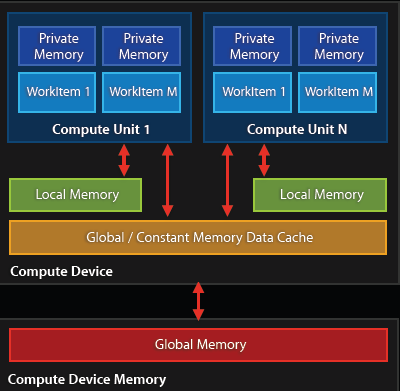Diagram of the memory hierarchy in a typical GPU. Credit: AMD.

In the previous code examples we’d always read and write from global memory, but every time we load something from there we will have to wait hundreds of clock cycles for it to be loaded. So it would be better if we’d load the initial data from global memory, used shared memory to store intermediate results and then store the final result back in global memory, to be read by the host. Let’s look at a program which blurs an image by dividing it into blocks, loading each block into a compute unit’s shared memory and having each core blur one specific pixel.

__kernel void blur(__global uchar4 *c,
__global uchar4 *res,
__local uchar4 *c_loc,
uint w, uint h)
{
uint xg = get_global_id(0);
uint yg = get_global_id(1);
uint xl = get_local_id(0)+1;
uint yl = get_local_id(1)+1;
uint wm = get_local_size(0)+2;
uint wl = get_local_size(0);
uint hl = get_local_size(1);
c_loc[xl+wm*yl] = c[xg+w*yg];
uint left = clamp(xg-1, (uint)0, w);
if(xl==1) c_loc[0+wm*yl] = c[left+w*yg];
uint right = clamp(xg+1, (uint)0, w);
if(xl==wl) c_loc[(wl+1)+wm*yl] = c[right+w*yg];
uint top = clamp(yg-1, (uint)0, h);
if(yl==1) c_loc[xl+wm*0] = c[xg+w*top];
uint bot = clamp(yg+1, (uint)0, h);
if(yl==hl) c_loc[xl+wm*(hl+1)] = c[xg+w*bot];
barrier(CLK_LOCAL_MEM_FENCE);
uchar4 blr = c_loc[xl+wm*(yl-1)]/(uchar)5 +
c_loc[xl-1+wm*yl]/(uchar)5 +
c_loc[xl+wm*yl]/(uchar)5 +
c_loc[xl+1+wm*yl]/(uchar)5 +
c_loc[xl+wm*(yl+1)]/(uchar)5;
res[xg+w*yg] = blr;
}


Whoa that’s a lot of lines! But they are all super simple, so let’s look at it line by line. In the function declaration you can see the __local uchar4 pointer. That points to the shared memory we are going to use. Unfortunately we cannot initialize it from the host (can only copy values to global memory) so we use lines 13-21 to read values from global memory into the local buffer, taking into account boundary conditions. In this code we distributed the threads in a 2d configuration so each thread has an id in both x and y dimensions (notice the argument for get_global_id and get_local_id denoting the dimension) so we can read off the x and y positions we want to process plus the block size directly in lines 6-12.

Once the block values have been copied to shared memory, we tell all the threads within one compute unit to wait for each other with line 22. This makes sure that everyone has loaded their corresponding values into shared memory before the code continues. It’s important to do this because each thread will read many values in the following computation. Line 23 is just the Laplace kernel which does the actual blurring. Finally we write the value back into global memory.

So how do we set up this code in pyopencl. I won’t reproduce the full code here, so let’s just look at the few important lines:

n_local = (16,12)
nn_buf = cl.LocalMemory(4*(n_local+2)*(n_local+2))
n_workers = (cat.size, cat.size)

prg.blur(queue, n_workers, n_local, pix_buf, pixb_buf, nn_buf, np.uint32(cat.size), np.uint32(cat.size))


LocalMemory is how we tell pyopencl we are going to use some shared memory in our kernel: we need to tell it how many bytes to reserve for this particular buffer. In this particular case we need block width plus two for the boundaries multiplied by block height plus boundaries, times 4 bytes for the size of a uchar4. n_workers corresponds to a tuple with the picture’s width and height, which means we launch a thread for each pixel in the image, distributed in the aforementioned 2d array. n_local corresponds to the local block size, i.e. how many threads will share a compute unit/shared memory. I encourage you to look at the full code and run it!

Next time I’ll cover some practical algorithms we can run on a gpu.